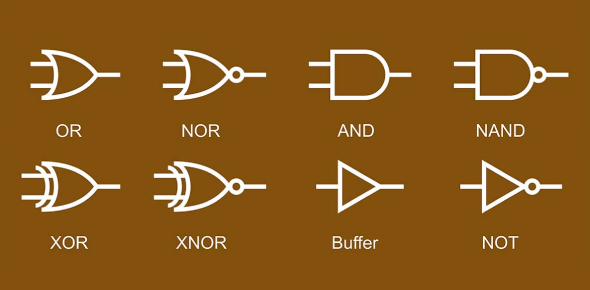# Boolean Algebra With The Logic Gates

25 Questions | Attempts: 2468
ShareSettings.

• 1.
Which gate only has an output of 1 if both inputs are 1?
• A.

OR

• B.

NAND

• C.

NOR

• D.

AND

• 2.
Which gate has an output of 1 if one but not both of the inputs is 1?
• A.

OR

• B.

XOR

• C.

NOT

• D.

AND

• 3.
Which gate has an output of 1 unless both inputs are 1?
• A.

NAND

• B.

AND

• C.

XOR

• D.

NOR

• 4.
Logic gates must have two inputs and one output
• A.

True

• B.

False

• 5.
XOR gates can be made from
• A.

All NAND gates

• B.

All NOR gates

• C.

All NOT gates

• D.

AND, OR and NOT gates

• 6.
NOR gates can be represented in logic notation as [Blank]
• 7.
It is possible to build up any logic gate using only NAND gates
• A.

True

• B.

False

• 8.
In boolean algebra, which operation does putting a bar above a letter represent
• A.

AND

• B.

NOT

• C.

OR

• D.

None of the above

• 9.
In boolean algebra, which operation does a + represent
• A.

OR

• B.

AND

• C.

NOT

• D.

None of the above

• 10.
What is a logic circuit?
• A.

A logic gate with three inputs and two outputs

• B.

A combination of logic gates whose output is always the same as its input

• C.

A combination of logic gates that carry out a particular function

• D.

A combination of logic gates whose output is always the same regardless of input

• 11.
If both inputs are 1 in an OR gate the output will be 0
• A.

True

• B.

False

• 12.
When constructing truth tables, you have to consider every last possible output
• A.

True

• B.

False

• 13.
Which gate gives outputs which are opposite to the outputs given by a NAND gate?
• A.

AND

• B.

OR

• C.

NOR

• D.

NOT

• 14.
Which gate will only output 1 if both inputs are 0?
• A.

NOR

• B.

OR

• C.

XOR

• D.

NAND

• 15.
Logic circuits cannot have more than 2 inputs
• A.

True

• B.

False

• 16.
Logic gates take in binary inputs and produce a [Blank] output
• 17.
To make your output the inverse of your input you can use the [Blank] gate
• 18.
The logic notation for a XOR gate is[Blank]
• 19.
Hexadecimal is a base [Blank] system
• 20.
Convert the hexadecimal A to binary
• A.

0001

• B.

1010

• C.

1111

• D.

1001

• 21.
A hexadecimal digit is equivalent to four binary digits
• A.

True

• B.

False

• 22.
What is a memory dump?
• A.

Unused storage space

• B.

A USB that can contain up to 200 petabytes

• C.

• D.

When memory contents are output to a monitor or printer

• 23.
Which logic gate can be represented by a triangle with a circle on one of its points?
• A.

NAND

• B.

OR

• C.

AND

• D.

NOT

## Related TopicsBack to top
×

Wait!
Here's an interesting quiz for you.